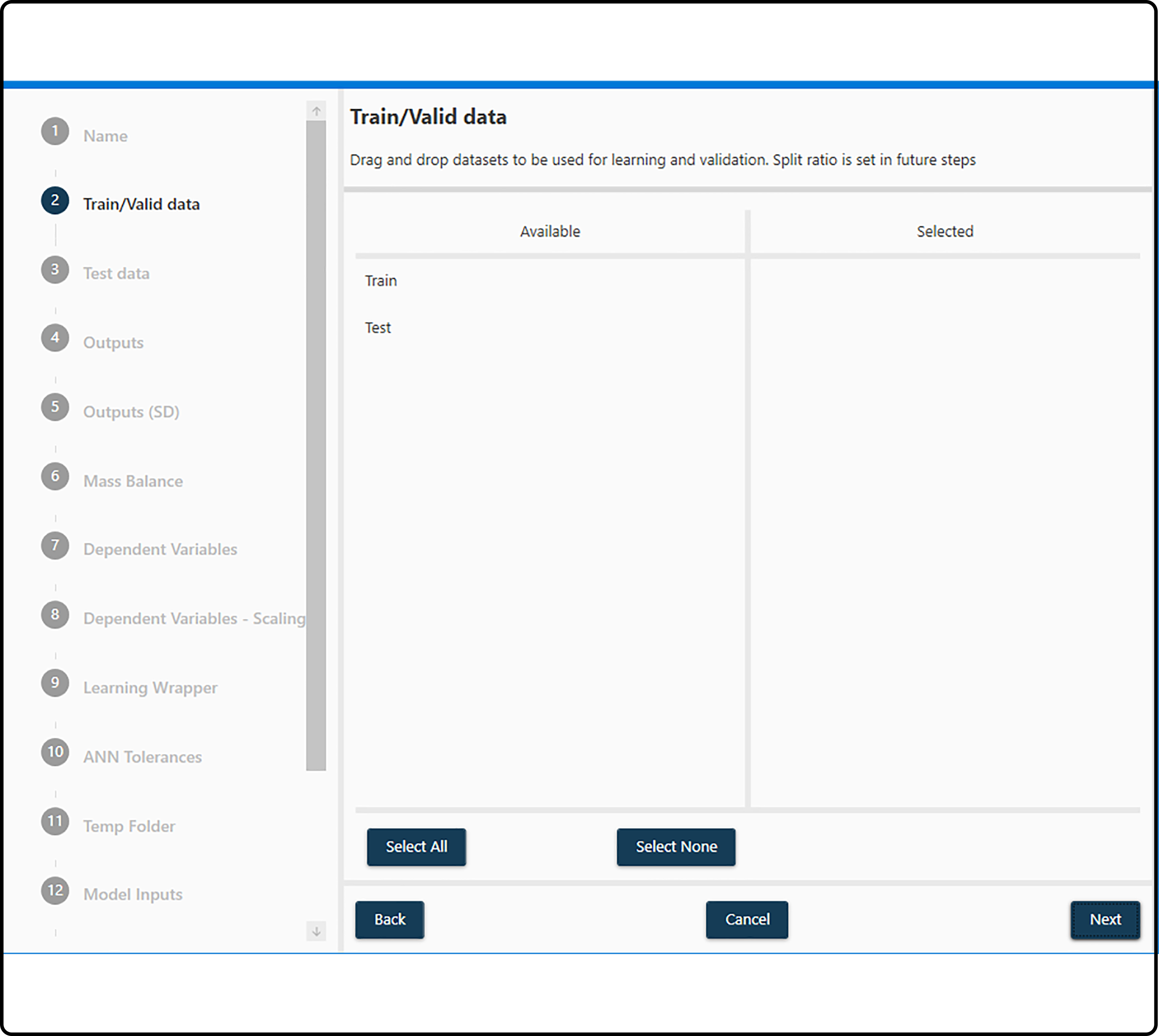# Datasets¶

## Types of data sets¶

Two kind of datasets need to be provided for setting up a hybrid model:

• Train/Validation. Dataset(s) used for training and validation purposes.

• Test. Dataset(s) used for testing.

Note

Training is the process of estimating/calculating model parameters, such as the neural network weights $$w$$ and biases $$b$$ (this process is often also called fitting a model). Typically, a model will have some (or even many) degrees of freedom regarding the model complexity. For a neural network model these are e.g. the number of hidden layers, the number of hidden units (per layer) or the number of iterations for weight updates. To identify a set of proper parameter values (with respect to the overall model performance), another data set is required (the so-called validation set) with no overlap with the training data set. Due to a limited amount of data in many cases, the data are often (repeately) splitted in training and a validation part. For data splitting see the section splitting ratio.

Note

The Test set is another data set (with no oberservations in common with the training or validation set), which is used to estimate the performance of one or a few final models. The resulting error measure (test error) gives us an idea, how the model will approximately perform in future experiments.

## Requirements¶

• All datasets being selected, no matter if they are intended for training, validation or for testing must have the same structure, i.e. the same number of variables (columns) with the exactly same name, so they can virtually be merged, compared…

Error

Not following this requirements will result in a model,

• not being able to be generated if it’s being created.

• not being able to start learning if it’s already generated but datasets where changed.

• All Output variables must contain a valid numeric value at the first row. If a dataset contains multiple runs (subsets), this requirement applies for each of these runs/subsets.

• All datasets must contain a Time variable (its actual name is not important), as the hybrid model is an integrative predictive model.

## Selection¶

• Datasets to be used for training/validation/testing can be selected by dragging and dropping from the left (Available) to the right side (Selected).

• Multiple datasets can be selected for each use type and will be virtually merged when needed.

• The same datasets can be selected for training/validation and testing. This might be necessary, if no test data are available.Figure 19. Selecting a data set (here: for training/validation) by dragging and dropping from the left to the right sub-window.

Caution

If the same datasets are selected for model setup and optimization (training/validation) and for testing the model, the estimated test error will underestimate the true test error, in some cases even dramatically. In this case, the validation error can be used as a surrogate for the test error (but is not as reliable as the latter and will still underestimate the true generalization error of the model). Therefore, it is strongly recommended to have a separate and independent data set for testing the model.